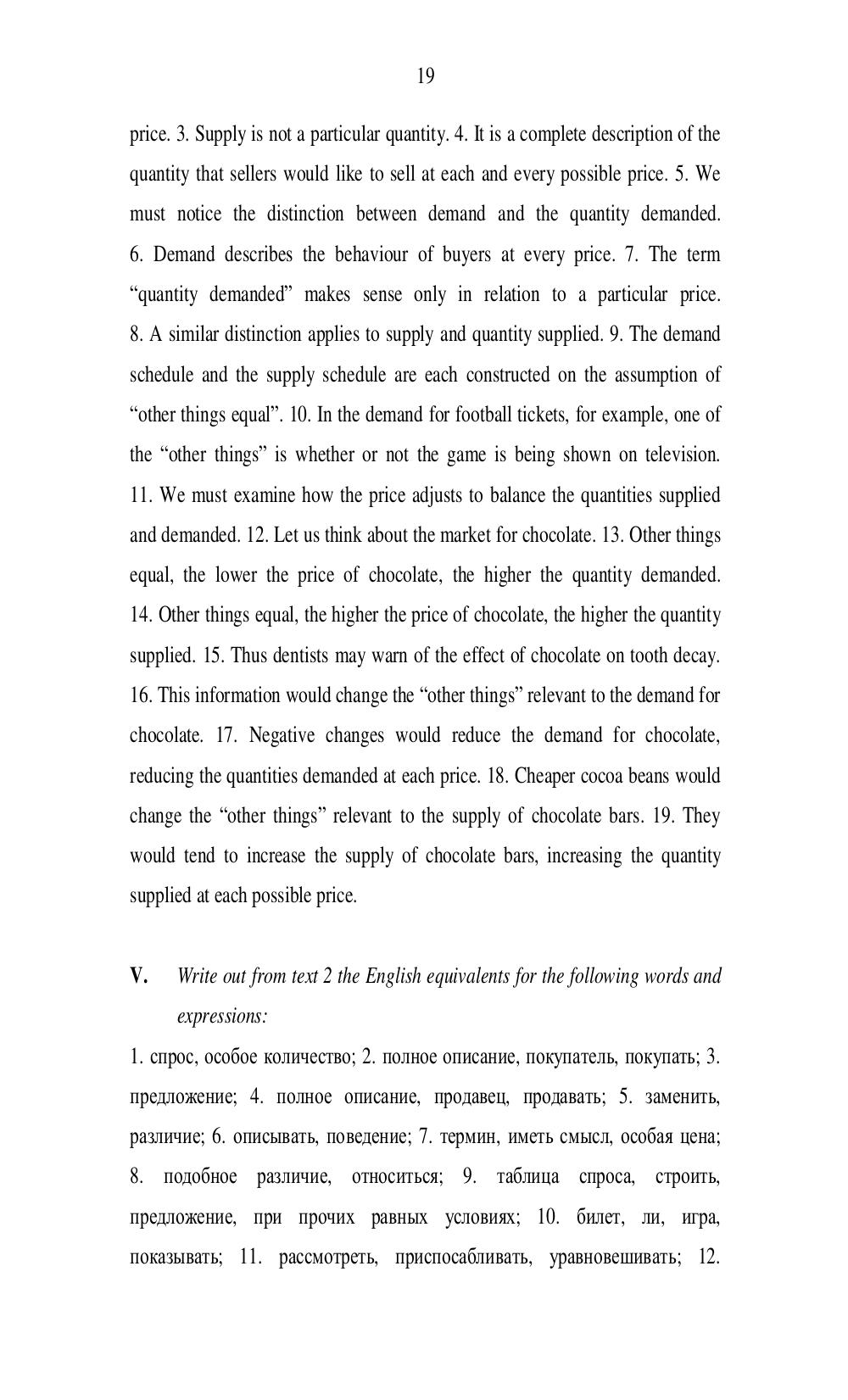# Английский язык. Агафонова И.Г - 19 стр.

Составители:

Рубрика:

• ## Иностранный язык

19
price. 3. Supply is not a particular quantity. 4. It is a complete description of the
quantity that sellers would like to sell at each and every possible price. 5. We
must notice the distinction between demand and the quantity demanded.
6. Demand describes the behaviour of buyers at every price. 7. The term
quantity demanded makes sense only in relation to a particular price.
8. A similar distinction applies to supply and quantity supplied. 9. The demand
schedule and the supply schedule are each constructed on the assumption of
other things equal. 10. In the demand for football tickets, for example, one of
the other things is whether or not the game is being shown on television.
11. We must examine how the price adjusts to balance the quantities supplied
and demanded. 12. Let us think about the market for chocolate. 13. Other things
equal, the lower the price of chocolate, the higher the quantity demanded.
14. Other things equal, the higher the price of chocolate, the higher the quantity
supplied. 15. Thus dentists may warn of the effect of chocolate on tooth decay.
16. This information would change the other things relevant to the demand for
chocolate. 17. Negative changes would reduce the demand for chocolate,
reducing the quantities demanded at each price. 18. Cheaper cocoa beans would
change the other things relevant to the supply of chocolate bars. 19. They
would tend to increase the supply of chocolate bars, increasing the quantity
supplied at each possible price.
V. Write out from text 2 the English equivalents for the following words and
expressions:
1. спрос, особое количество ; 2. полное описание , покупатель, покупать ; 3.
предложение ; 4. полное описание , продавец, продавать ; 5. заменить ,
различие ; 6. описывать , поведение ; 7. термин, иметь смысл, особая цена ;
8. подобное различие , относиться; 9. таблица спроса , строить ,
предложение , при прочих равных условиях; 10. билет, ли , игра,
показывать ; 11. рассмотреть, приспосабливать , уравновешивать ; 12.19

price. 3. Supply is not a particular quantity. 4. It is a complete description of the
quantity that sellers would like to sell at each and every possible price. 5. We
must notice the distinction between demand and the quantity demanded.
6. Demand describes the behaviour of buyers at every price. 7. The term
“quantity demanded” makes sense only in relation to a particular price.
8. A similar distinction applies to supply and quantity supplied. 9. The demand
schedule and the supply schedule are each constructed on the assumption of
“other things equal”. 10. In the demand for football tickets, for example, one of
the “other things” is whether or not the game is being shown on television.
11. We must examine how the price adjusts to balance the quantities supplied
and demanded. 12. Let us think about the market for chocolate. 13. Other things
equal, the lower the price of chocolate, the higher the quantity demanded.
14. Other things equal, the higher the price of chocolate, the higher the quantity
supplied. 15. Thus dentists may warn of the effect of chocolate on tooth decay.
16. This information would change the “other things” relevant to the demand for
chocolate. 17. Negative changes would reduce the demand for chocolate,
reducing the quantities demanded at each price. 18. Cheaper cocoa beans would
change the “other things” relevant to the supply of chocolate bars. 19. They
would tend to increase the supply of chocolate bars, increasing the quantity
supplied at each possible price.

V.    Write out from text 2 the English equivalents for the following words and
expressions:
1. спрос, особое количество; 2. полное описание, покупатель, покупать; 3.
предложение; 4. полное описание, продавец, продавать; 5. заменить,
различие; 6. описывать, поведение; 7. термин, иметь смысл, особая цена;
8.   подобное     различие,    относиться;     9.   таблица     спроса,    строить,
предложение, при прочих равных условиях; 10. билет, ли, игра,
показывать; 11. рассмотреть, приспосабливать, уравновешивать; 12.